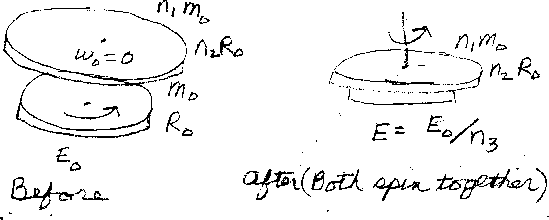Problem D7: Initially, a disk is spinning. The disk has a mass of m0 and a radius of R0. The kinetic energy of rotation that it initially has is E0. Then, another disk (initially not rotating) is placed on top of the first one. The second disk has a mass of n1m0 and a radius of n2R0. The axes of the two disks are in line. There is friction between the two disks, and the two disks end up spinning at the same rate. What is the final kinetic energy of rotation of the two disks? If the final rotational energy of the system is E = E0/n3, what is n3? Note: n1, n2, and n3 are unitless.n1 = n2 = Input n3:
If you are currently in my class, you can record your grade by entering your name and student ID number (without the leading zeros) below and clicking on "record grade".
 First Name = Last Name = ID = Problem: